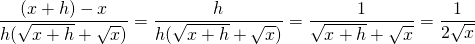# Solution assignment 04 The basics

### Assignment 4

Calculate the value of the following fraction as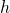approaches to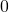: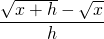### Solution

If we substitute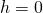we get an undefined result. Therefore we use a trick: we multiply both numerator and denominator by: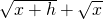and get: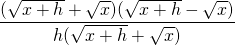We rewrite the numerator by applying one of the special products and finally we can safely substitute: